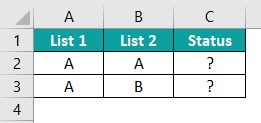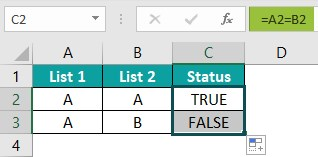# Compare Two Lists In ExcelArticle byExcelMojo Team## What Is Compare Two Lists In Excel?

The Compare Two Lists feature in Excel is a powerful tool enabling users to compare two data lists and identify their differences. This tool is particularly useful when working with large datasets, as it saves time and reduces errors that are likely to occur when comparing lists manually.

This example consists of two lists we will compare using the equal sign operator.

Let us compare between the two lists using this formula.

The formula entered is =A2=B2; We can see the result in cell C2.

###### Key Takeaways
• Comparing two Excel lists can help us save time and increase efficiency when working with data-based projects.
• Utilizing the techniques above to compare two lists in Excel is contingent upon the data structure. It is imperative to employ the appropriate methodology to ensure accuracy and efficiency.
• If the data is not properly organized, row-by-row matching may not be the most suitable approach.
• Effective data organization is crucial for accurate analysis and interpretation. When data is disorganized, matching rows one-by-one may not yield the desired results.
• It is important to ensure data is appropriately structured before matching or analysis.

### How To Compare Two Lists In Excel?

When comparing two lists in Excel, it is crucial to ensure that the data in the columns match exactly.

First, sort both lists alphabetically or numerically to identify and locate differences easily. Next, highlight one column and navigate to the Conditional Formatting section under the Home tab.

Select Highlight Cells Rules, then Duplicate Values. This will highlight any duplicate values in the selected column. Repeat this same step for the second list.

By doing so, we can quickly identify which cells are missing from either list or if there are any differences between the matching values.

#### #1 – Compare Two Lists Using Equal Sign Operator

The example contains two lists; we compare them using the equal sign operator.

To calculate a value using an Excel formula, it is important to follow these steps:

Step 1: Select the cell where we will enter the formula. For this example, let us choose cell C2.

Step 2: Input the complete formula =A2=B2 into cell C2.

Step 3: Press the Enter key.

We can see the result as FALSE in cell C2. To proceed, press Enter and drag the cursor to cell C8, as shown in the image. We will obtain the desired outcome by inputting the formula correctly and pressing Enter after each value.

#### #2 – Match Data By Using Row Difference Technique

This example features two lists, and we utilize the Row Difference Technique to match data. To accurately calculate a value using an Excel formula, it is crucial to adhere to the following steps:

Step 1: Choose the cells with data. In this example, let us select cell range A2:B8.

Step 2: Press the F5 key on the keyboard, and the Go to: window opens; select the Special button from the window.

Step 3: Next, choose the Row differences option from the Go to Special window, and then click on the OK button.

Step 4: The cells are highlighted as shown in the below image.

Step 5: Now, let us change the color of these cells.

#### #3 – Match Row Difference By Using IF Condition

This example showcases utilizing two lists and the Match Row Difference technique through an IF condition.

To ensure precise calculation of values using an Excel formula, it is essential to follow the steps outlined below:

Step 1: Select the cell where we will enter the formula. For this example, let us choose cell C2.

Step 2: Input the complete formula =IF(A2=B2, “Matched,” “Not Matched”) into cell C2.

Step 3: Press the Enter key. The resulting value in cell C2 will be Not Matched, as depicted in the accompanying image. To proceed, press Enter and drag the cursor to cell C8, as demonstrated in the subsequent image.

It is crucial to adhere to these steps precisely to ensure accurate results. We can obtain the desired outcome by inputting the formula correctly and pressing Enter after each value.

#### #4 – Match Data Even If There Is A Row Difference

This example serves as a demonstration of the effective utilization of two lists and the Data, Even If There is a Row Difference, achieved through the use of a VLOOKUP excel function.

To ensure the precise calculation of values using an Excel formula, it is imperative to follow the steps outlined below:

Step 1: Select the cell where we will enter the formula. For this example, let us choose cell E2.

Step 2: Input the complete formula =VLOOKUP(C2, \$A\$2:\$A\$7,1,0) into cell E2.

Step 3: Press the Enter key. The resulting value in cell E2 will be the #N/A error, as depicted in the accompanying image. To proceed, press Enter and drag the cursor to cell E7.

We can obtain the desired outcome by inputting the formula correctly and pressing Enter after each value. These steps ensure the calculation is performed accurately and efficiently, providing reliable results.

Step 4: We will utilize the IFERROR excel function to rectify the error.

Step 5: Next, input the entire formula =IFERROR (VLOOKUP (C2, \$A\$2:\$A\$7,1,0), “Not Available”) into cell E2. Remember to press the Enter key after entering each value in the previous step. The resulting value in cell E2 will be FALSE, as illustrated in the accompanying image.

To proceed, press Enter and drag the cursor to cell E7, as shown in the below image. This will enable us to resolve the error.

#### #5 – Highlight All The Matching Data

This example demonstrates the effective utilization of two lists and Excel’s “Highlight All the Matching Data” feature.

To ensure accurate calculation of values using an Excel formula, it is important to follow the below steps:

Step 1: Select the cells where the contents will be displayed. In this particular illustration, cells A2:B7 will be chosen. Select the Duplicate Values option from the Conditional Formatting, Highlight Cells Rules option under the Home tab.

Step 2: The Duplicate values box opens;

Select the option and click OK. The duplicate cells will be highlighted.

Step 3: To highlight the unique cells again, open the Duplicate values box opens, make the change as shown below and click OK. The unique cells will be highlighted.

Both the duplicate and unique cells are highlighted in different tables.

#### #6 – Partial Matching Technique

This example help us learn Partial Matching using VLOOKUP in Excel.

To ensure precise calculation of values using this formula, it is imperative to follow the steps outlined below:

Step 1: Select the cell where we will enter the formula. For this example, let us choose cell E2.

Step 2: Input the complete formula =VLOOKUP(“*”&D2&“*”, \$A\$2:\$B\$6,2,0) into cell E2.

Step 3: Press the Enter key. We can see the result in cell E2.

Next, press Enter and drag the cursor to cell E7, as shown in the below image.

### Important Things To Note

• Formulas, such as COUNTIF or VLOOKUP, allow for more customization and potential for automation of the comparison process and are used to Compare Two Lists in Excel.
• When working with large amounts of Excel data, comparing two lists is essential. There are several ways to do this, but one of the most straightforward and effective methods involves using the VLOOKUP function.
• The VLOOKUP function searches for a value in one list and returns a corresponding value from another, making it easy to identify differences between the two data sets.
• Excel also offers conditional formatting options allowing us to highlight cells automatically based on specific criteria.

1. What is the purpose of comparing two lists in Excel?

The purpose of comparing two lists in Excel is
* To identify any differences or similarities between the two data sets.
* Dealing with large data sets, as it enables users to quickly and efficiently analyze and manipulate information.
* By comparing lists, Excel can highlight similar or different values between the two lists, allowing users to identify changes or discrepancies.

Ultimately, this functionality simplifies data analysis and enables users to make informed decisions based on their findings.

2. Are there any limitations or drawbacks to comparing lists in Excel?

While Excel is a highly effective tool for comparing lists, there are still some limitations and drawbacks. One primary concern with comparing Excel lists is the potential for overlooking human data entry errors. This can result in inaccurate comparisons, particularly when columns must be sorted or filtered for more granular analysis. Additionally, while Excel allows for the customization of comparison criteria, achieving the desired results can require extensive time and effort. The software’s capabilities may also limit those without advanced Excel skills and need external programs or add-ins to complete more thorough comparisons. While Excel is a valuable tool for list comparison, it’s important to proceed cautiously and remain aware of its limitations during usage.

3. How do we compare two lists in Excel?

This particular example comprises two lists, which we shall compare using the equal sign operator.The formula entered is =A2=B2; the result is shown below.